## 3.2.2 One-Dimensional POISSON's Equation

As an example the discretized form of POISSON's equation (2.14) will be written using the finite difference method. Assuming constant permittivity POISSON's equation in the one-dimensional case is given by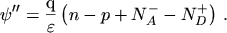(3.11)

The discretized representation is obtained by replacing the second order derivative by the central difference quotient eqn. (3.10) at all inner points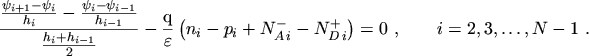(3.12)

The equations for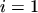and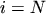are determined by the boundary conditions. By rewriting this equation in the form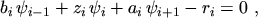(3.13)

the coefficients are found to be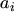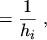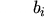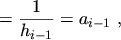(3.14)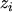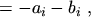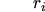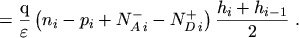(3.15)

The coefficient matrix resulting from the linear equation system eqn. (3.13) is symmetric and only the diagonal and the secondary diagonal are filled with elements different from zero.

M. Gritsch: Numerical Modeling of Silicon-on-Insulator MOSFETs PDF